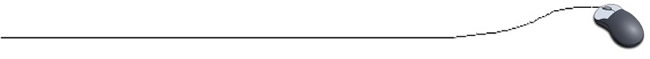Simple Animation

The process of simple animation is a series of events where a figure appears (is drawn), disappears (is erased in some manner), and reappears (is redrawn) in another location. As this process continues, the figure appears to be moving. Since a computer can draw and erase a figure faster than we can see it happen, we must be able to control the time that a figure remains viewable on the screen to accomplish a simple animation.

 A Timer: (to create a PAUSE in the screen display)
We will take a look at two possible timers that will cause the screen to pause.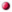a timing method to be added to your program
public static void pause (int time)
{
try
//opens an exception handling statement
{
}
catch(InterruptedException e)
//captures the exception
{
}
}

Note: Thread.sleep(5000) will sleep for approximate 5 seconds (a long time).a Timer class that exists within Swing is utilized
This timer works in conjunction with an ActionListener.
An ActionListener is an "event handler" that defines what should be done when a user performs a certain operation. When dealing with event handlers, it is necessary to use import java.awt.event.*;

We know that import java.awt.*; imports classes which belong to this specific package. But there are sub-packages of AWT that will not be included in this import statement.
import java.awt.event.*; is a separate package which defines classes and interfaces used for event handling in AWT and Swing.

(snippets of code to show insertions) . . .
public class Demo extends JPanel implements ActionListener
{
Timer time = new Timer(5, this); // 'this' refers to the ActionListener
. . .
public void paintComponent(g)
{
time.start(); //engage the timer
. . .

If time is a variable of type Timer, then the statement
time = new Timer(millisDelay,listener);
creates a timer with a delay of millisDelay milliseconds between events (where 1000 milliseconds equals one second).Establishing an Animation "Formula"

The process of moving a figure on the screen necessitates knowing the coordinate locations of the various positions of the figure on the screen. It is too cumbersome to code every single location. Instead, the figure is expressed using one or more variables that are accessed by the computer to position and reposition the figure on the screen.

If the figure that you are moving is one of the simple primitive shapes that are already method-defined, such as simple rectangles and circles, the animation "formula" process is quite simple.Animation Formulas for existing method-defined figures (draw or fill):
Consider this rectangle: g.drawRect(35, 45, 25, 35);
• To move the rectangle horizontally, we use g.drawRect(x, 45, 25, 35);
Replace the x-coordinate with a variable to move horizontally.
• To move the rectangle vertically, we use g.drawRect(35, y, 25, 35);
Replace the y-coordinate with a variable to move vertically.
• To move the rectangle diagonally, we use g.drawRect(x, y, 25, 35);
Replace the x and y coordinates with variables to move diagonally.
Then instruct the computer to change the values of x (and/or y) in a manner that meets the speed and direction of the desired movement.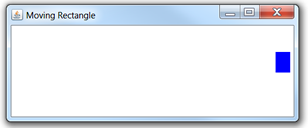Rectangle moved horizontally across screen.

The process of erasing a figure is accomplished with repaint( ); which calls the paintComponent method. It tells the system that a component needs to be redrawn. Think of it as refreshing the viewing area.

Animations require the use of an ActionListener. Remember that an ActionListener is an "event handler" which requires the import statement import java.awt.event.*;
The event handler class specifies that the class will implement an ActionListener interface:
public class ClassName extends JPanel implements ActionListener {
The following method is needed to implement the action listener interface every 5 milliseconds (in this example):
public void actionPerformed(ActionEvent e) {

//Program to Move Rectangle Across the JPanel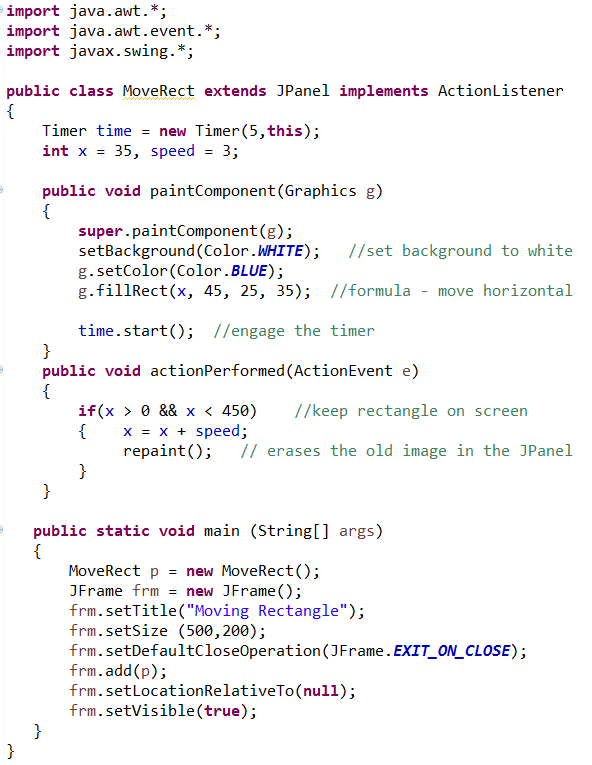Animation Formulas are a bit harder for method-defined polygons:
Consider the arrow figure shown below. Yes, this can be a method-defined 7-sided polygon since all of its sides are line segments. The first box shows the coordinates of where we want the arrow to be located when it starts. We will animate the arrow moving horizontally to the right.

 Figure in Starting Position: Figure Written with Formula: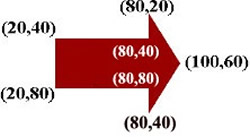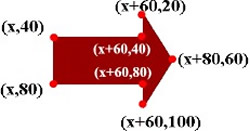Record the coordinates of the figure in its starting position before the animation begins. int [ ] a = {20, 80, 80, 100, 80, 80, 20}; int [ ] b = {40, 40, 20, 60, 100, 80, 80}; g.fillPolygon(a, b, 7); This arrow is going to move horizontally to the right.  The smallest x-value is 20.  The number 20 is replaced with x, and all other x coordinates are written in terms of x = 20. int [ ] a = {x, x+60, x+60, x+80, x+60, x+60, x}; int [ ] b = {40, 40, 20, 60, 100, 80, 80}; g.fillPolygon(a, b, 7); It was decided for this arrow that the x-values would range from a starting value of 20 to an ending value of 310 with the figure being redrawn every 3 pixels.